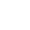﻿

/    2019-04-15

三. 类的其他成员

3.1 类方法

``class Student:    __num = 0    def __init__(self,name,age):        self.name = name        self.age= age        Student.addNum()  # 写在__new__方法中比较合适，但是现在还没有学，暂且放到这里    @classmethod    def addNum(cls):        cls.__num += 1    @classmethod    def getNum(cls):        return cls.__numa = Student('太白金星', 18)b = Student('武sir', 36)c = Student('alex', 73)print(Student.getNum())``

3.2 静态方法

``import timeclass TimeTest(object):    def __init__(self, hour, minute, second):        self.hour = hour        self.minute = minute        self.second = second    @staticmethod    def showTime():        return time.strftime("%H:%M:%S", time.localtime())print(TimeTest.showTime())t = TimeTest(2, 10, 10)nowTime = t.showTime()print(nowTime)``

3.3 属性

property是一种特殊的属性，访问它时会执行一段功能（函数）然后返回值

``例一：BMI指数（bmi是计算而来的，但很明显它听起来像是一个属性而非方法，如果我们将其做成一个属性，更便于理解）成人的BMI数值：过轻：低于18.5正常：18.5-23.9过重：24-27肥胖：28-32非常肥胖, 高于32　　体质指数（BMI）=体重（kg）÷身高^2（m）　　EX：70kg÷（1.75×1.75）=22.86``

``class People:    def __init__(self,name,weight,height):        self.name=name        self.weight=weight        self.height=height    @property    def bmi(self):        return self.weight / (self.height**2)p1=People('egon',75,1.85)print(p1.bmi)例一代码``

``class Foo:    @property    def AAA(self):        print('get的时候运行我啊')    @AAA.setter    def AAA(self,value):        print('set的时候运行我啊')    @AAA.deleter    def AAA(self):        print('delete的时候运行我啊')#只有在属性AAA定义property后才能定义AAA.setter,AAA.deleterf1=Foo()f1.AAAf1.AAA='aaa'del f1.AAA或者：class Foo:    def get_AAA(self):        print('get的时候运行我啊')    def set_AAA(self,value):        print('set的时候运行我啊')    def delete_AAA(self):        print('delete的时候运行我啊')    AAA=property(get_AAA,set_AAA,delete_AAA) #内置property三个参数与get,set,delete一一对应f1=Foo()f1.AAAf1.AAA='aaa'del f1.AAA``
``class Goods(object):    def __init__(self):        # 原价        self.original_price = 100        # 折扣        self.discount = 0.8    @property    def price(self):        # 实际价格 = 原价 * 折扣        new_price = self.original_price * self.discount        return new_price    @price.setter    def price(self, value):        self.original_price = value    @price.deltter    def price(self, value):        del self.original_priceobj = Goods()obj.price         # 获取商品价格obj.price = 200   # 修改商品原价del obj.price     # 删除商品原价商品实例商品示例``

四. isinstace 与 issubclass

``class A:    passclass B(A):    passobj = B()print(isinstance(obj,B))print(isinstance(obj,A))isinstance``

isinstance(a,b)：判断a是否是b类（或者b类的派生类）实例化的对象

``class A:    passclass B(A):    passclass C(B):    passprint(issubclass(B,A))print(issubclass(C,A))issubclass``

ssubclass(a,b)： 判断a类是否是b类（或者b的派生类）的派生类

``from collections import Iterableprint(isinstance([1,2,3], list))  # Trueprint(isinstance([1,2,3], Iterable))  # Trueprint(issubclass(list,Iterable))  # True# 由上面的例子可得，这些可迭代的数据类型，list str tuple dict等 都是 Iterable的子类。``(0)

﻿